ML Wiki

Sequenced Queries

Temporal Databases provide mechanisms to store and manipulate time-varying information. But how to query it?

Valid Time Tables

A valid time table is a table with

• attributes that specify a period when the information in these tables were valid
• in SQL92 it's usually modeled with 2 date attributes from and to

For example,

• Employee(SSN, FirstName, LastName, BirthDate)
• Position(PCN, JobTitle)
• Incumbents(SSN, PCN, FromDate, ToDate)
• Salary(SSN, Amount, FromDate, ToDate)
• Incumbents and Salary are valid-time tables

Example

Consider this table:

Incumbents

SSN PCN FromDate ToDate
111223333 900225 1996-01-01 1996-06-01
111223333 900225 1996-06-01 1996-08-01
111223333 900225 1996-08-01 1996-10-01
111223333 900225 1996-10-01 3000-01-01
111223333 900225 1997-01-01 3000-01-01

This is a valid time table:

• it has attributes from and to that show when this information is valid
• special date 3000-01-01 is used to show "currently valid" (i.e. till now)
• Closed-open periods are typically used:
• it makes it easier to merge in SQL: just do $T_1.\text{to} = T_2.\text{from}$

Type of Queries

On Valid-Time tables we can query for:

• current state: now
• time-sliced: valid at some point of time
• sequenced: applied to each point in time
• non-sequenced: applied to all points in time, completely ignoring temporal aspects

Temporal Keys and Constraints

Consider this table again:

Incumbents

SSN PCN FromDate ToDate
111223333 900225 1996-01-01 1996-06-01
111223333 900225 1996-06-01 1996-08-01

What are keys that we can use to uniquely identify a record in this table?

• candidate keys:
• (SSN, PCN, FromDate)
• (SSN, PCN, ToDate)
• (SSN, PCN, FromDate, ToDate)

But we want to enforce the following constraint:

• An employee can have only one position at a point of time
• none of these keys can enforce such a constraint

Sequenced Primary Key

This can only be enforced with triggers

 CREATE TRIGGER Seq_Primary_Key ON Incumbents FOR INSERT, UPDATE AS IF EXISTS (SELECT * FROM Incumbents AS I1 -- (1) no overlap WHERE 1 < (SELECT COUNT(I2.SSN) FROM Incumbents AS I2 WHERE I1.SSN = I2.SSN AND I1.PCN = I2.PCN -- <= the key AND I1.FromDate < I2.ToDate AND I2.FromDate < I1.ToDate)) OR EXISTS -- no NULL (SELECT * FROM Incumbents AS I WHERE I.SSN IS NULL OR I.PCN IS NULL) BEGIN RAISERROR('Violation of sequenced primary key constraint',1,2) rollback transaction END We look for intervals with the same key that overlap with each over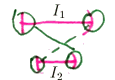also we don't allow NULLs

Other Constraints

And there are other constraints that we want to enforce:

• No Duplicates
• Referential Integrity (introducing sequenced foreign keys)
• The history must be contiguous (no gaps)

Queries

Coalescing

Consider this table:

• Employee(Name, Salary, Title, BirthDate, FromDate, ToDate)
• each time the salary or title change, the time of the change is captured and new record with this change is added
• so we can see the entire history of changes
Name Salary Title BirthDate FromDate ToDate
John 60.000 Assistant 9/9/60 1/1/95 1/6/95
John 70.000 Assistant 9/9/60 1/6/95 1/10/95
John 70.000 Lecturer 9/9/60 1/10/95 1/2/96
John 70.000 Professor 9/9/60 1/2/96 1/1/97

We see how the salary grows and how John changes his title

Now consider these queries:

• show John's current salary
• show the history of John's salary changes

sequenced queries

The first query is easy:

SELECT Salary
FROM Employee
WHERE Name = 'John' AND
FromDate <= now() AND
now() <= ToDate

But it's not easy to do the second one

Main idea:

• find intervals that overlap or adjacent and merge them
•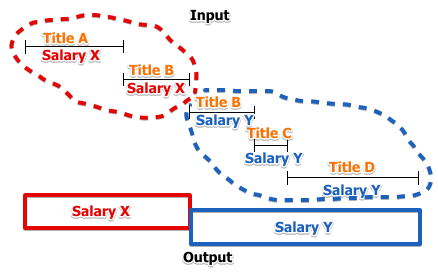• if we have 5 periods
• (title A, salary X), (title B, salary X), (title B, salary Y), (title C, salary Y), (title D, salary Y)
• we want to extract only periods with equal salary and get (salary X) and (salary Y)

Imperative Version

Idea:

• create a temporary table and modify the timestaps of intervals there
• for period $T_1$ find a period $T_2$ for which
• they have the same salary
• they are adjacent or overlap
• $T_1$ starts before $T_2$ starts
• $T_2$ starts when $T_1$ has not finished yet
• $T_2$ finishes after $T_1$ finishes
•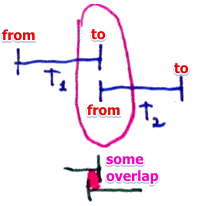• if there exists such $T_2$ then update the finish time of $T_1$ to $T_2$
•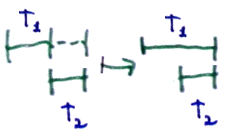CREATE TABLE Temp(Salary, FromDate, ToDate) AS
SELECT Salary, FromDate, ToDate FROM Employee
WHERE Name = 'John'
repeat
UPDATE TEMP T1
SET (T1.ToDate) =
(SELECT MAX(T2.ToDate)
FROM TEMP AS T2
WHERE T1.Salary = T2.Salary
AND T1.FromDate < T2.FromDate
AND T1.ToDate >= T2.FromDate
AND T1.ToDate < T2.ToDate) WHERE EXISTS
(SELECT *
FROM TEMP AS T2
WHERE T1.Salary = T2.Salary
AND T1.FromDate < T2.FromDate
AND T1.ToDate >= T2.FromDate
AND T1.ToDate < T2.ToDate)
until no tuples updated

This is how it looks like after each pass:

•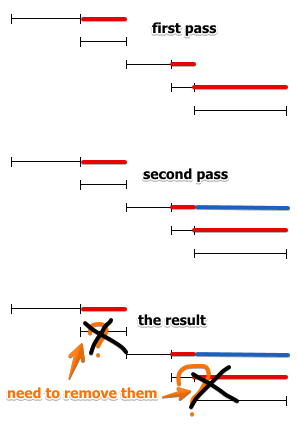• we see that it there are intervals that are no longer needed
• so we remove intervals that are not maximal

Removal of non-maximal intervals

• for interval $T_1$ find interval $T_2$ such that
• it has the same salary
• $T_2$ includes $T_1$:
• $T_2$ start after $T_1$ and
• $T_2$ finish earlier than $T_1$
• td-coalecsing-imperative-remove-min.png
• if there exists such $T_2$ then remove $T_1$

DELETE FROM Temp T1
WHERE EXISTS (
SELECT * FROM Temp AS T2
WHERE T1.Salary = T2.Salary AND
((T1.FromDate > T2.FromDate  AND T1.ToDate <= T2.ToDate) OR
(T1.FromDate >= T2.FromDate AND T1.ToDate <  T2.ToDate))

Declarative Version in SQL

Algorithm:

Let $E$ be a database with John's history

• for salary $x$ we need to find $f, l \in E$ such that
• $f$ is the first period with salary $x$ and $l$ last period with $x$
• and the interval between $f$ and $l$ is continuous:
• for all $t \in E$ with salary $x$ that inside $[f, l)$
• there's $t_1 \in E$ that starts before $t$ and finishes before $t$ finishes
• to ensure that $f,l$ are indeed the bounds of the interval we try to find $t_2 \in E$ such that
• it either starts before $f$ or finishes after $l$
• if we find such $t_2$ - $[f, l)$ is not maximal and we need to try another pair of $f,l$

More formally this looks like this:

find $f, l \in E$ such that
$f.\text{from} < l.\text{to} \land f.\text{salary} = l.\text{salary} \land$
$\forall t \in E$
$t.\text{salary} = f.\text{salary} \land f.\text{from} < t.\text{from} \land t.\text{from} < l.\text{to}:$
$\exists t_1 \in E: t_1.\text{salary} = f.\text{salary} \land$
$t_1.\text{from} < t.\text{from} \land f.\text{from} \leqslant t1.\text{to}$
$\land$
$\lnot \exists t_2 \in E$
$t_1.\text{salary} = f.\text{salary} \land$
$\Big[ (t_2.\text{from} < f.\text{from} \land f.\text{from} \leqslant t_2.\text{to}) \lor$
$\ (t_2.\text{from} \leqslant l.\text{to} \land l.\text{to} < t_2.\text{to}) \Big]$

Let's see how it looks like graphically

 find $f, l \in E$ such that $f.\text{from} < l.\text{to} \land$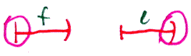$f.\text{salary} = l.\text{salary} \land$ $\forall t \in E$ $\ \ t.\text{salary} = f.\text{salary} \land$ $\ \ f.\text{from} < t.\text{from} \land t.\text{from} < l.\text{to} \land$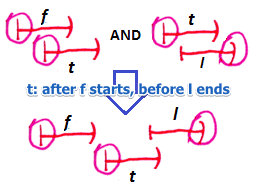$\ \ \exists t_1 \in E:$ for all such $t$ there exists $t_1$ $\ \ \ \ t_1.\text{salary} = f.\text{salary} \land$ $\ \ \ \ t_1.\text{from} < t.\text{from} \land f.\text{from} \leqslant t1.\text{to}$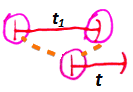$\land$ $\lnot \exists t_2 \in E$ $\ \ t_1.\text{salary} = f.\text{salary} \land$ $\ \ \Big[ (t_2.\text{from} < f.\text{from} \land f.\text{from} \leqslant t_2.\text{to}) \lor \\ \ \ \ (t_2.\text{from} \leqslant l.\text{to} \land l.\text{to} < t_2.\text{to}) \Big]$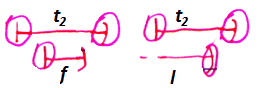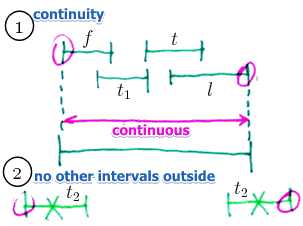But there's no $\forall$ operator in SQL:

• so need to replace the condition $\forall t ... \exists t_1$ onto $\not \exists t ... \not \exists t_1$

Here's a SQL version:

CREATE VIEW Temp(Salary, FromDate, ToDate) AS
SELECT Salary, FromDate, ToDate
FROM Employee
WHERE Name = 'John';

SELECT DISTINCT F.Salary, F.FromDate, L.ToDate
FROM Temp AS F, Temp AS L
WHERE F.FromDate < L.ToDate
AND F.Salary = L.Salary
-- SUBQUERY 1: ensure continuity
AND NOT EXISTS
(SELECT * FROM Temp AS T
WHERE T.Salary = F.Salary
AND F.FromDate < T.FromDate
AND T.FromDate < L.ToDate
AND NOT EXISTS
(SELECT * FROM Temp AS T1
WHERE T1.Salary = F.Salary
AND T1.FromDate < T.FromDate
AND T.FromDate <= T1.ToDate))
-- SUBQUERY 2: make sure it's maximal
AND NOT EXISTS
(SELECT * FROM Temp AS T2
WHERE T2.Salary = F.Salary
AND ((T2.FromDate < F.FromDate AND F.FromDate <= T2.ToDate)
OR (T2.FromDate <= L.ToDate AND L.ToDate < T2.ToDate)));

Temporal Join

Alternative solution:

• the schema before had two independent attributed we want to capture
• can't we reorganize the schema - split the information about changes in salary and title?

So we split and have this schema:

• Employee(Name, BirthDate)
• EmployeeSal(Name, Salary, FromDate, ToDate)
• EmployeeTitle(Name, Title, FromDate, ToDate)

Showing the history of changes in John's salary is easy now:

SELECT Salary, FromDate, ToDate
FROM EmployeeSal
WHERE Name = 'John'

However how we now obtain a table of salary and title intervals?

• we need a temporal join

EmployeeSal

Name Salary FromDate ToDate
John 60.000 1/1/95 1/6/95
John 70.000 1/6/95 1/1/97

EmployeeTitle

Name Title FromDate ToDate
John Assistant 1/1/95 1/10/95
John Lecturer 1/10/95 1/2/96
John Professor 1/2/96 1/1/97

EmployeeSal $\Join$ EmployeeTitle

Name Salary Title FromDate ToDate
John 60.000 Assistant 1/1/95 1/6/95
John 70.000 Assistant 1/6/95 1/10/95
John 70.000 Lecturer 1/10/95 1/2/96
John 70.000 Professor 1/2/96 1/1/97

The idea:

• to find overlapping intervals and join on them
• there are 4 possible ways in which intervals can overlap
• so we need to try all of them
 -- (1) SELECT S.Name, Salary, Title, S.FromDate, S.ToDate FROM EmployeeSal S, EmployeeTitle T WHERE S.Name = T.Name AND T.FromDate <= S.FromDate AND S.ToDate <= T.ToDate UNION ALL -- (2) SELECT S.Name, Salary, Title, S.FromDate, T.ToDate FROM EmployeeSal S, EmployeeTitle T WHERE S.Name = T.Name AND S.FromDate > T.FromDate AND T.ToDate < S.ToDate AND S.FromDate < T.ToDate UNION ALL -- (3) SELECT S.Name, Salary, Title, T.FromDate, S.ToDate FROM EmployeeSal S, EmployeeTitle T WHERE S.Name = T.Name AND T.FromDate > S.FromDate AND S.ToDate < T.ToDate AND T.FromDate < S.ToDate UNION ALL -- (4) SELECT S.Name, Salary, Title, T.FromDate, T.ToDate FROM EmployeeSal S, EmployeeTitle T WHERE S.Name = T.Name AND T.FromDate >= S.FromDate AND T.ToDate <= S.ToDate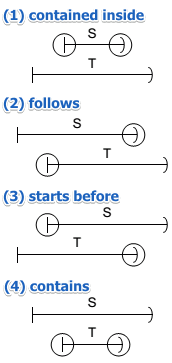Aggregation Functions

Suppose we want to apply some aggregation function to each period in the valid time table

Example:

• compute the maximal salary for each period
•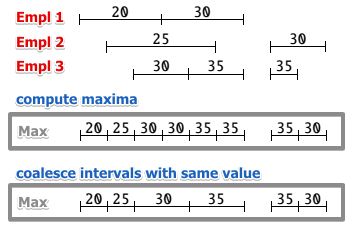This is done in 3 steps:

• compute all possible periods
• compute the maximal salary for these periods
• coalesce the periods with the same salary

Step 1:

-- all moments of time when there was some change
CREATE VIEW SalChanges(Day) as
SELECT DISTINCT FromDate
FROM Salary
UNION
SELECT DISTINCT ToDate
FROM Salary;

-- now we construct periods from these moments
CREATE VIEW SalPeriods(FromDate, ToDate) as
SELECT P1.Day, P2.Day
FROM SalChanges P1, SalChanges P2
WHERE P1.Day < P2.Day
AND NOT EXISTS
(SELECT * FROM SalChanges P3
WHERE P1.Day < P3.Day
AND P3.Day < P2.Day);

Step 2:

CREATE VIEW TempMax(MaxSalary, FromDate, ToDate) as
SELECT MAX(E.Amount), I.FromDate, I.ToDate
FROM Salary E, SalPeriods I
WHERE E.FromDate <= I.FromDate
AND I.ToDate <= E.ToDate
GROUP BY I.FromDate, I.ToDate;

Step 3

• coalesce these intervals

Count is a little bit different

• we need to identify gaps in the history and put there zeros
•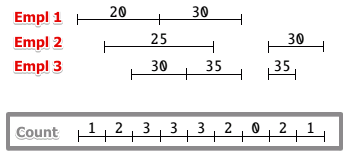Step 2 for count

CREATE VIEW TempCount(NbEmp, FromDate, ToDate) as
SELECT COUNT(*), P.FromDate, P.ToDate
FROM Salary S, SalPeriods P
WHERE S.FromDate <= P.FromDate
AND P.ToDate <= S.ToDate
GROUP BY P.FromDate, P.ToDate

UNION ALL

SELECT 0, P.FromDate, P.ToDate
FROM SalPeriods P
WHERE NOT EXISTS
(SELECT * FROM Salary S
WHERE S.FromDate <= P.FromDate
AND P.ToDate <= S.ToDate);